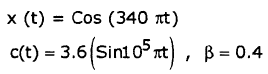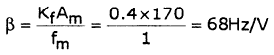Courses

# Test: Analog Communication - 1

## 15 Questions MCQ Test GATE ECE (Electronics) 2022 Mock Test Series | Test: Analog Communication - 1

Description
This mock test of Test: Analog Communication - 1 for Electronics and Communication Engineering (ECE) helps you for every Electronics and Communication Engineering (ECE) entrance exam. This contains 15 Multiple Choice Questions for Electronics and Communication Engineering (ECE) Test: Analog Communication - 1 (mcq) to study with solutions a complete question bank. The solved questions answers in this Test: Analog Communication - 1 quiz give you a good mix of easy questions and tough questions. Electronics and Communication Engineering (ECE) students definitely take this Test: Analog Communication - 1 exercise for a better result in the exam. You can find other Test: Analog Communication - 1 extra questions, long questions & short questions for Electronics and Communication Engineering (ECE) on EduRev as well by searching above.
QUESTION: 1

Solution:
QUESTION: 2

Solution:
QUESTION: 3

### If a FM transmitter radiates signal with power Pt and modulation index β then

Solution:
QUESTION: 4

Which of the following systems have less figure of merit?

Solution:
QUESTION: 5

Which of the following modulations is preferred for voice communication?

Solution:
QUESTION: 6

Which of the following system cannot achieve maximum power efficiency compared to others?

Solution:
QUESTION: 7

In a broadcast superheterodynes receiver having no RF amplifier, the loaded Q of the antenna coupling circuit is 100. If the intermediate frequency is 455 kHz. The image frequency at 1000 kHz will be

Solution:
*Answer can only contain numeric values
QUESTION: 8

The antenna current of an AM transmitter is 10 A when the carrier is alone transmitted and is increased to 11 A after modulation.

The power efficiency of the above AM transmitter is____________________ (%)

Solution: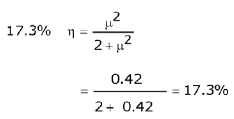*Answer can only contain numeric values
QUESTION: 9

Input to the matched filter is x(t),which is shown in figure. The value of impulse response of matched filter at t = 5.4 sec is__________________________________________________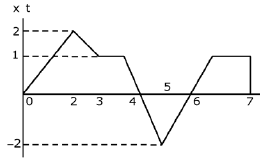Solution: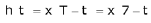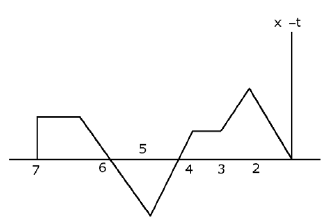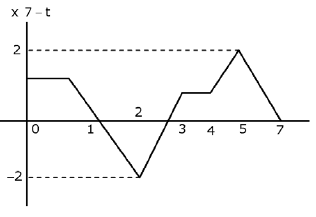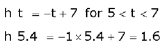QUESTION: 10

The set up shown below is used to convert a Narrow Band FM signal with modulation index = 0.6 to a wide Band FM Signal with frequency deviation equal to 600 KHz and carrier frequency of 12.5 MHz. Then determine nlr n2.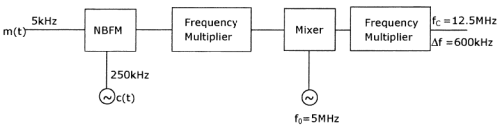Solution: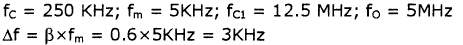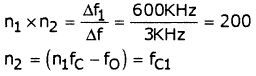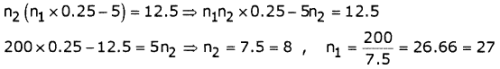QUESTION: 11

A single tone signal 14Cos(40π x103t) is used to modulate a carrier Accoswct. Then determine the number of channels available in very high frequency (VHF) band for this signal, if amplitude modulation and frequency modulation scheme employs respectively {Assume sensitivity of FM modulator = 10KHz/V}

Solution:

VHF band (30MHz - 300MHz)

Total Bandwidth available = 2700MHz

Bandwidth of AM signal =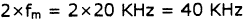Bandwidth of FM signal =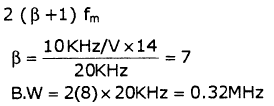Total number of channels available for AM Signal =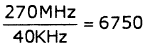for FM Signal =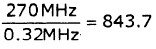QUESTION: 12

Determine Bandwidth of signal y(t) in the figure shown below.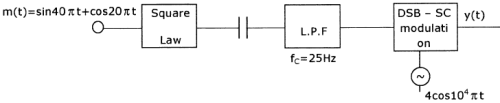Solution: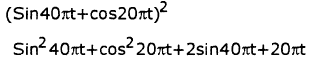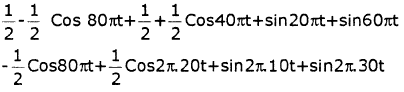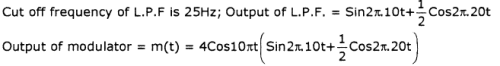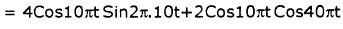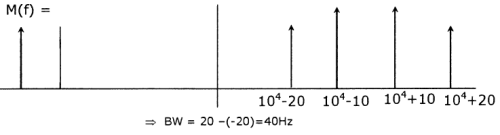QUESTION: 13

For the figure shown below, what should be minimum bandwidth of channel such that the signal x(t) & y(t) can be transmitted through channel simultaneously without any distortion?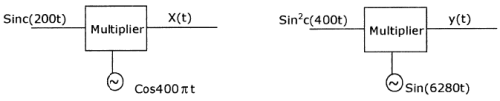Solution:

Minimum Bandwidth required = 1400-100 = 1300Hz

m1(t) = sinc1(200t) ;        = 200Hz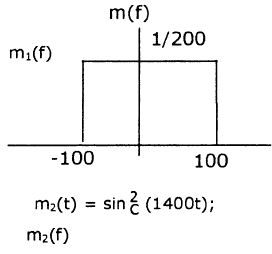fc2 = 1000Hz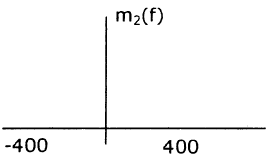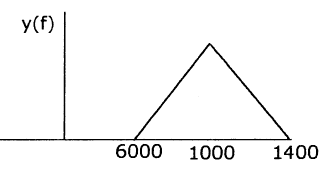QUESTION: 14

A carrier signal Accosukt is frequency modulated using a message signal 3cos103nt. If the signal to noise ratio at input and output of the receiver are found to be 20dB and 40dB respectively, then determine sensitivity of frequency modulator.

Solution: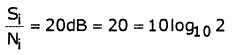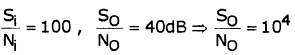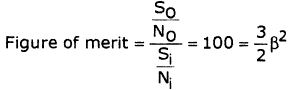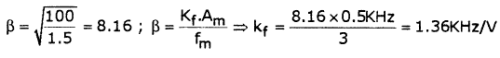QUESTION: 15

What will be sensitivity of modulator shown below, if its depth of modulation is

0.4 and x(t) = cos (340nt) ?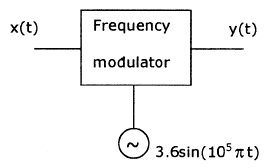Solution: